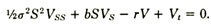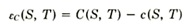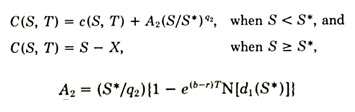Posted in Finance, Accounting and Economics Terms, Total Reads: 1021

## Definition: Barone-Adesi and Whaley Model

Also known as the quadratic approximation model, it is the American Version of Black- Scholes- Merton Model. The Black- Scholes model gives an estimation of European options. It is mainly utilized by the people who engage in options- trading. This model was first issued as the ‘Efficient Analytic Approximation of American Option Values’ by Giovanni Barone- Adesi and Robert E. Whaley. This model gives a simple approximation for price- exchanged American call and put options applied on commodities and futures based on commodities.

The assumptions of this model are as follows:

1. Short- term interest rate, r, and cost of carrying the commodity, b, are assumed constant and proportional rates

2. For non- dividend stock, cost of carrying equal to riskless rate i.e. b=r. According to Merton’s model, b<r for dividend- yield options

As b is assumed constant, the relation between futures and commodity prices is,

F= SebT

Where F, S= Current Futures and Spot prices respectively

T= Time of expiry of futures contract

According to Merton’s Model, the partial differential equation which explains the commodity option pricing discussion isWhere V determines the price of contingent claim.

To obtain the solution of this equation, the Barone Adesi and Whaley (BAW) Model divides the American price into two parts: The European Price and the early exercise premium (feature present only in an American option)Where Ɛc is the early exercise premium

C(S, T) = American Commodity option value and

C(S, T)= European Commodity option value

This equation will have to satisfy the partial differential equation

Here, C(S, T) is given by:The critical value of S* is obtained by solving the Newton’s algorithm of finding the root

By finding the value of C(S, T) and c(S, T), the partial differential equation can be solved and the American option- price can be determined

1. Accurate

2. Computationally efficient

3. Less Expensive than binomial or compound option pricing models

Hence, this concludes the definition of Barone-Adesi and Whaley Model along with its overview.

Browse the definition and meaning of more terms similar to Barone-Adesi and Whaley Model. The Management Dictionary covers over 7000 business concepts from 6 categories.

Search & Explore : Management Dictionary

Similar Definitions from same Category: